Скачать презентацию Chapter 20 Production and Costs economic costs

e1181dcdc4ac5558d0a5b67a4ac49cb3.ppt

• Количество слайдов: 49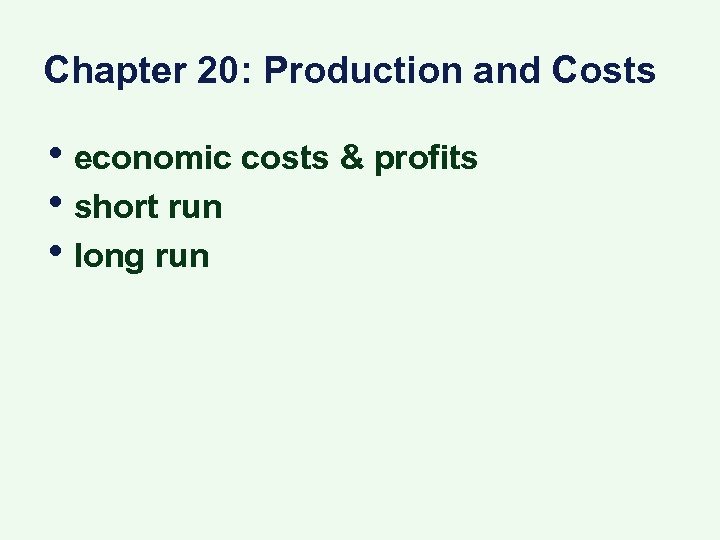Chapter 20: Production and Costs • economic costs & profits • short run • long run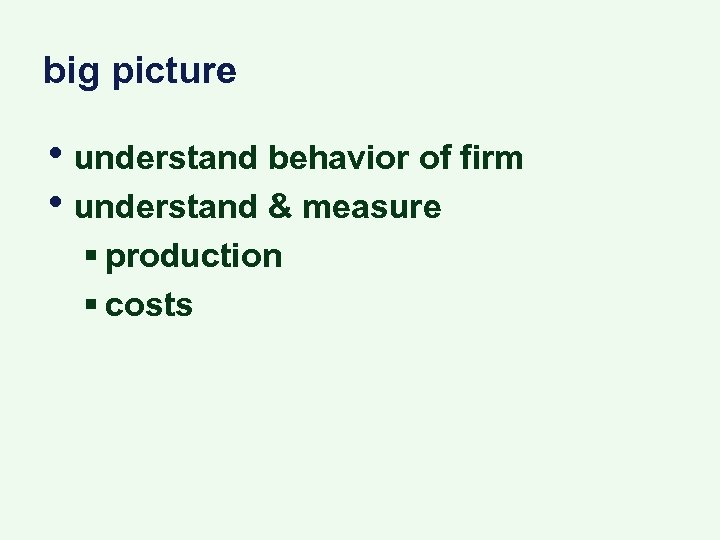big picture • understand behavior of firm • understand & measure § production § costs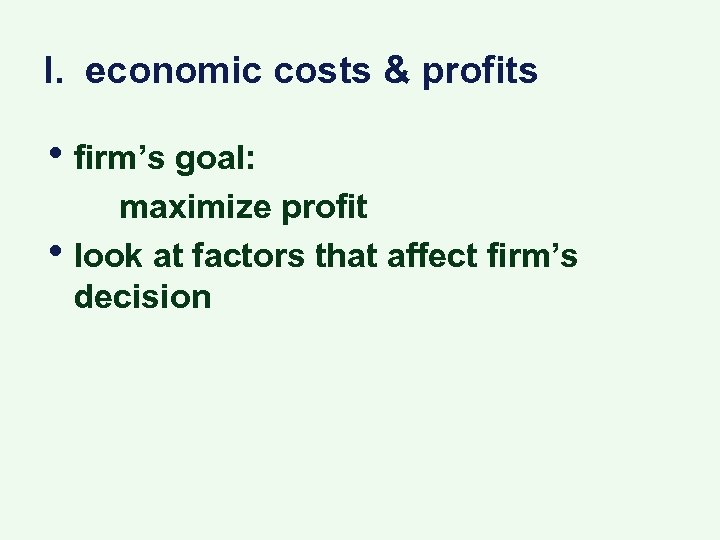I. economic costs & profits • firm’s goal: • maximize profit look at factors that affect firm’s decision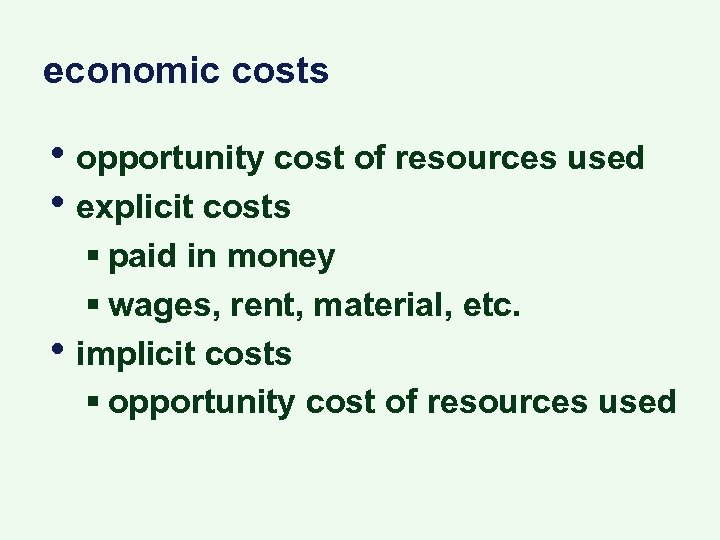economic costs • opportunity cost of resources used • explicit costs • § paid in money § wages, rent, material, etc. implicit costs § opportunity cost of resources used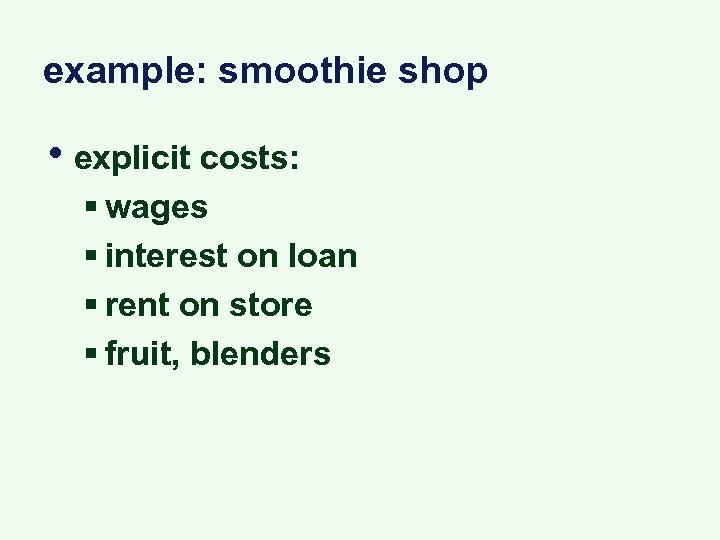example: smoothie shop • explicit costs: § wages § interest on loan § rent on store § fruit, blenders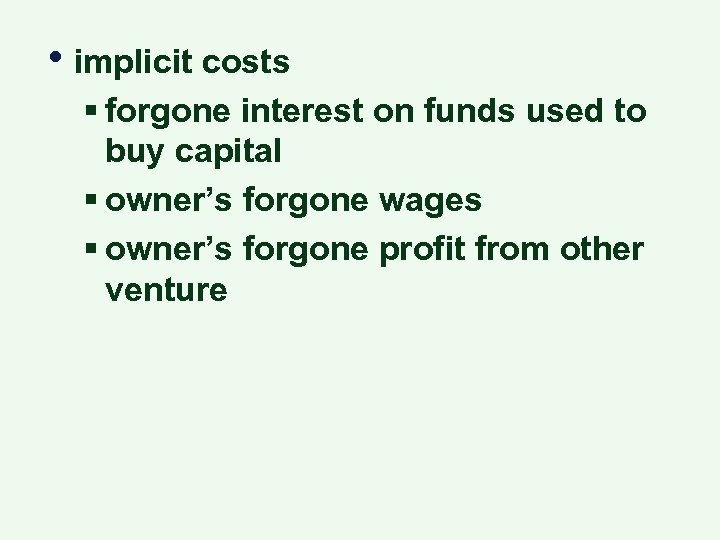• implicit costs § forgone interest on funds used to buy capital § owner’s forgone wages § owner’s forgone profit from other venture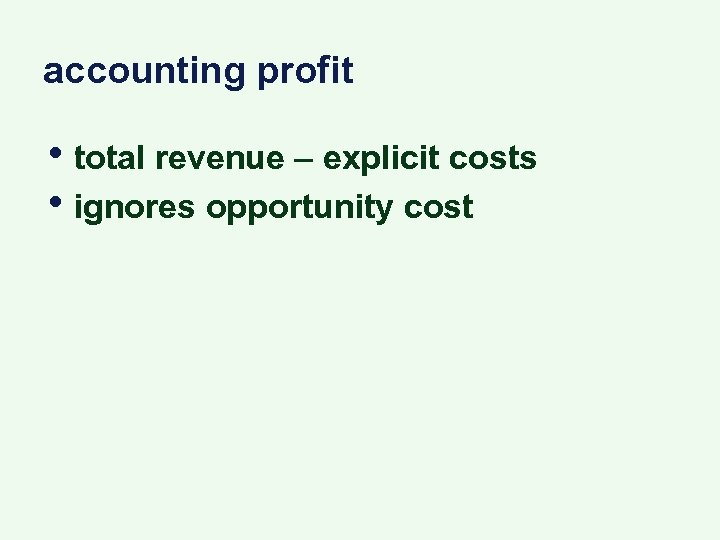accounting profit • total revenue – explicit costs • ignores opportunity cost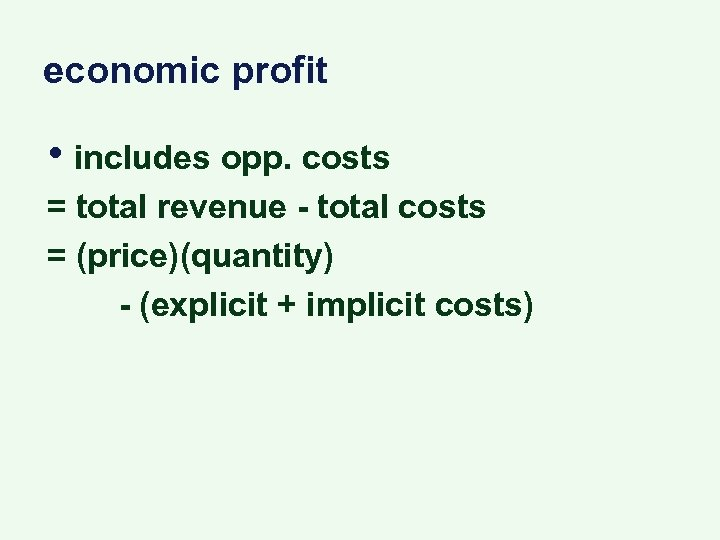economic profit • includes opp. costs = total revenue - total costs = (price)(quantity) - (explicit + implicit costs)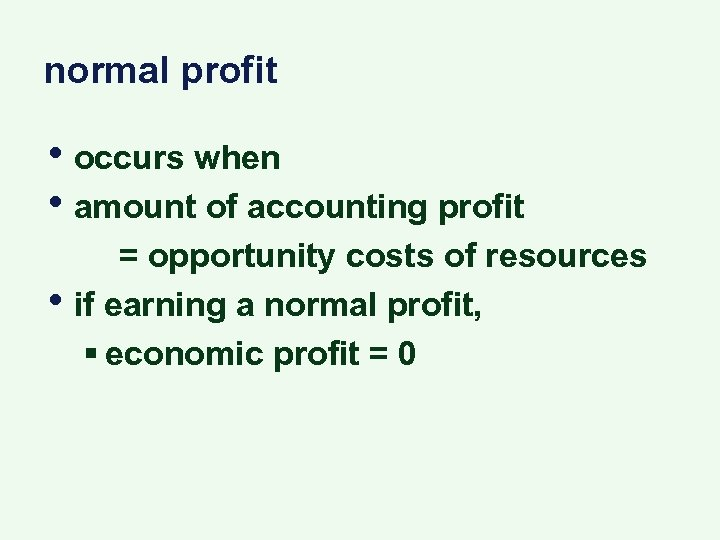normal profit • occurs when • amount of accounting profit • = opportunity costs of resources if earning a normal profit, § economic profit = 0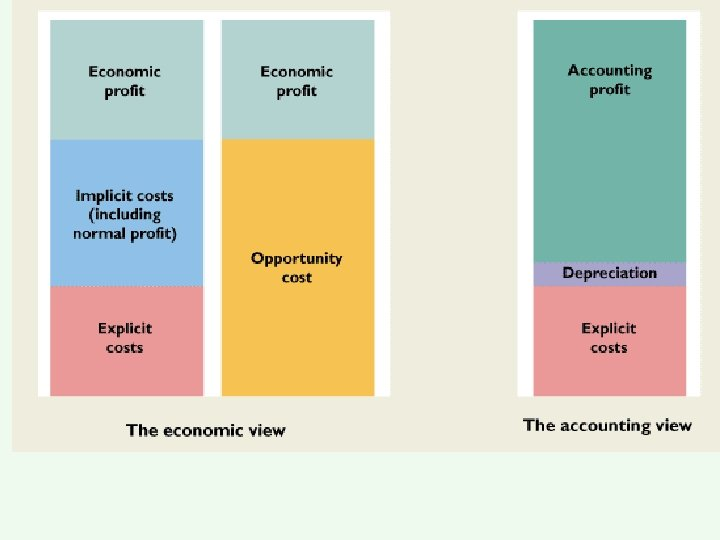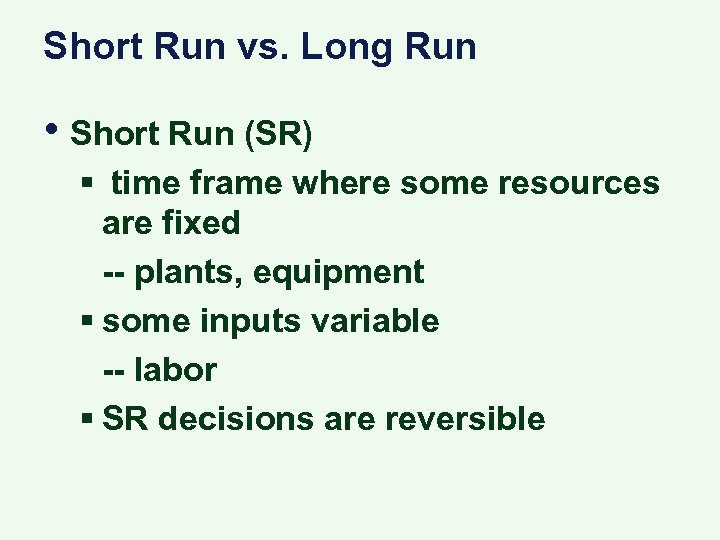Short Run vs. Long Run • Short Run (SR) § time frame where some resources are fixed -- plants, equipment § some inputs variable -- labor § SR decisions are reversible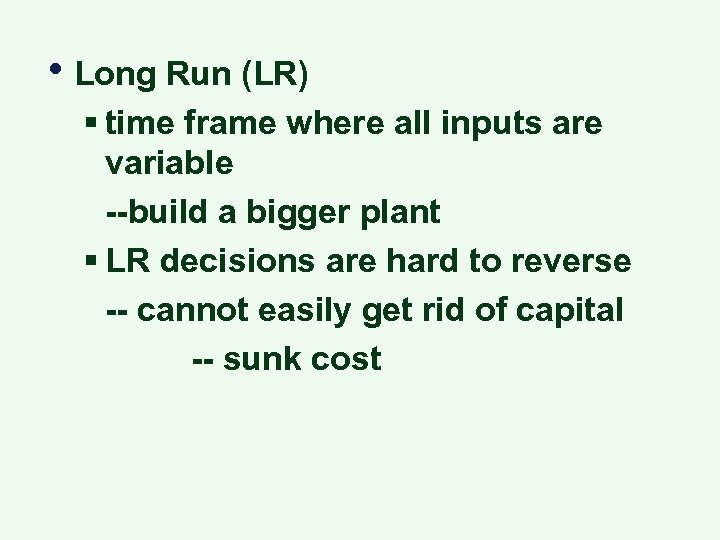• Long Run (LR) § time frame where all inputs are variable --build a bigger plant § LR decisions are hard to reverse -- cannot easily get rid of capital -- sunk cost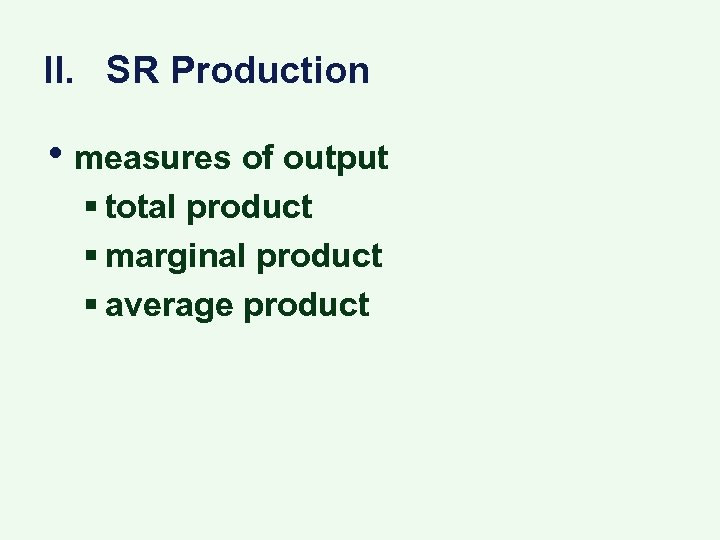II. SR Production • measures of output § total product § marginal product § average product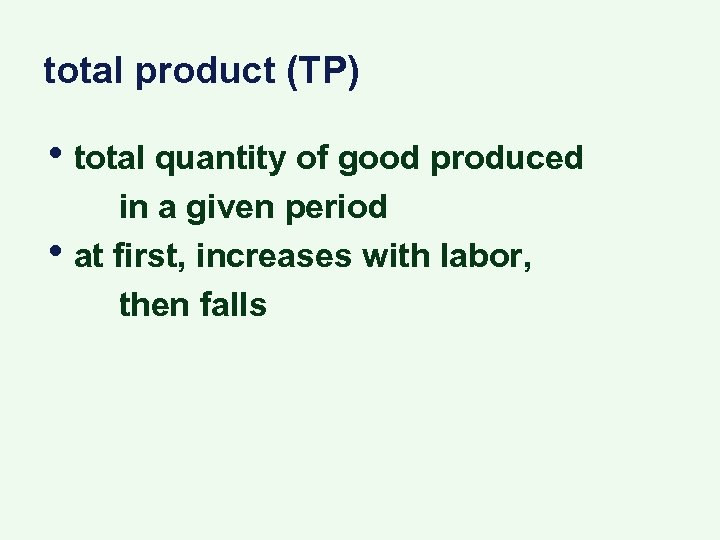total product (TP) • total quantity of good produced • in a given period at first, increases with labor, then falls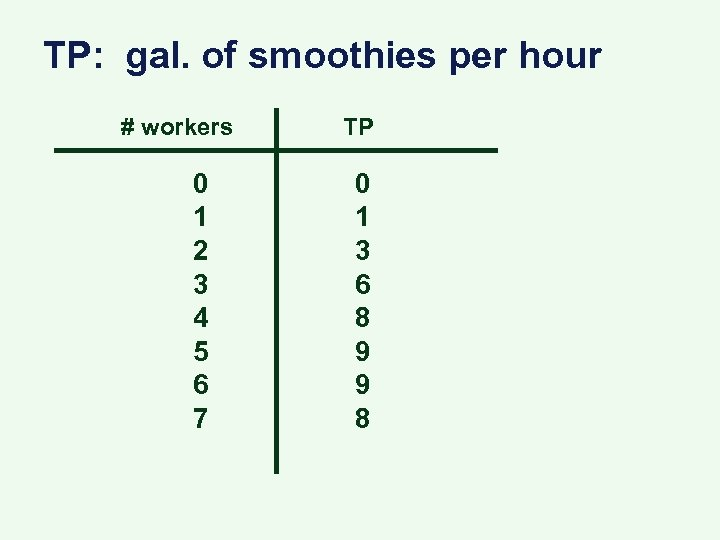TP: gal. of smoothies per hour # workers 0 1 2 3 4 5 6 7 TP 0 1 3 6 8 9 9 8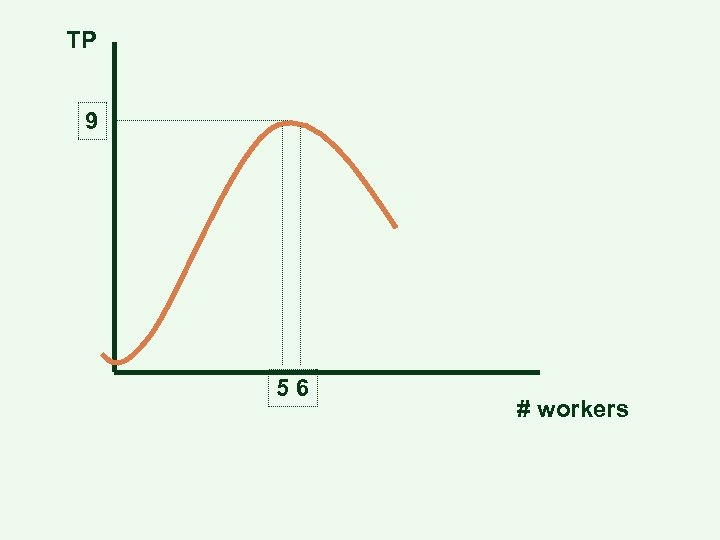TP 9 56 # workers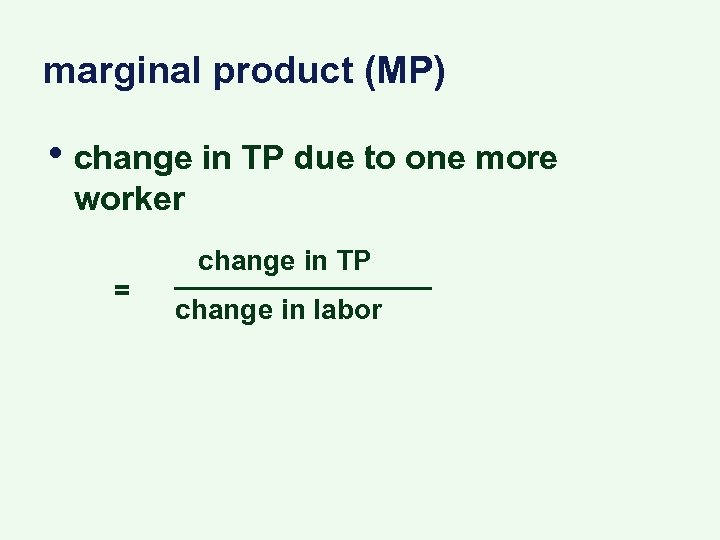marginal product (MP) • change in TP due to one more worker = change in TP change in labor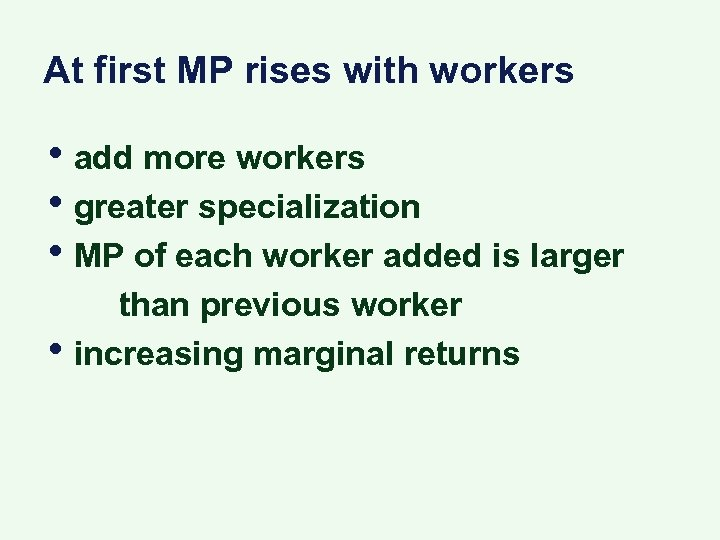At first MP rises with workers • add more workers • greater specialization • MP of each worker added is larger • than previous worker increasing marginal returns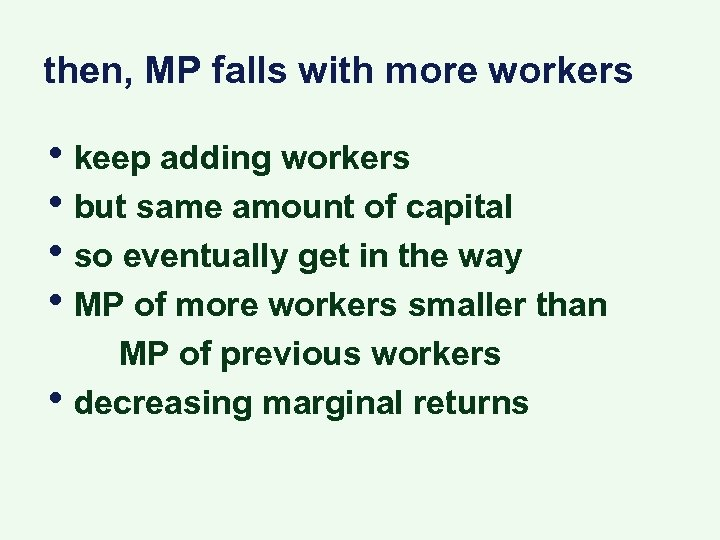then, MP falls with more workers • keep adding workers • but same amount of capital • so eventually get in the way • MP of more workers smaller than • MP of previous workers decreasing marginal returns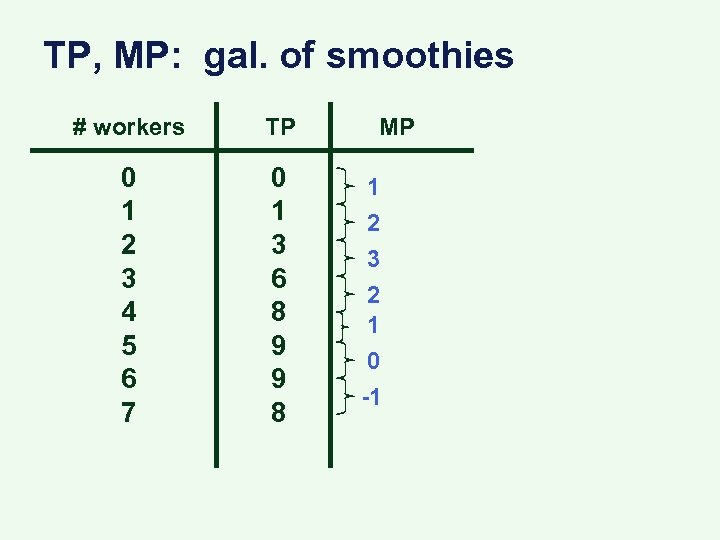TP, MP: gal. of smoothies # workers TP 0 1 2 3 4 5 6 7 0 1 3 6 8 9 9 8 MP 1 2 3 2 1 0 -1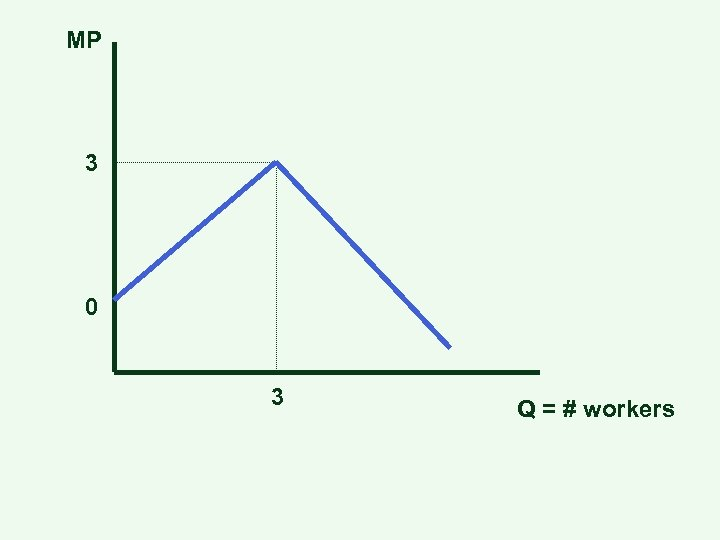MP 3 0 3 Q = # workers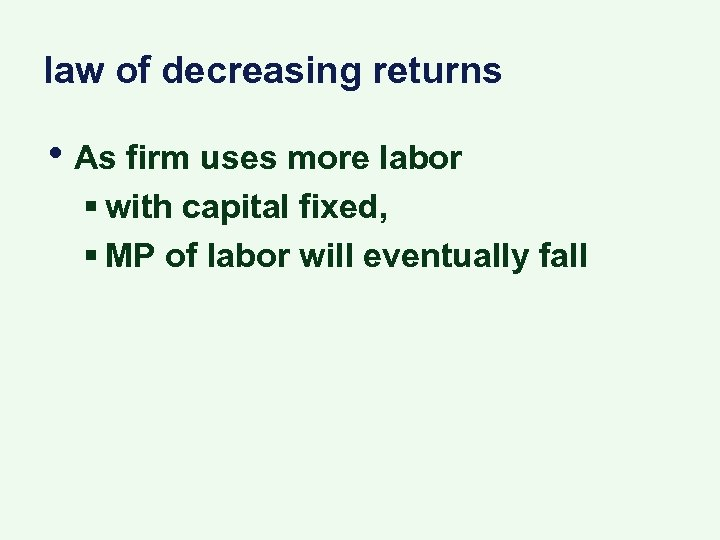law of decreasing returns • As firm uses more labor § with capital fixed, § MP of labor will eventually fall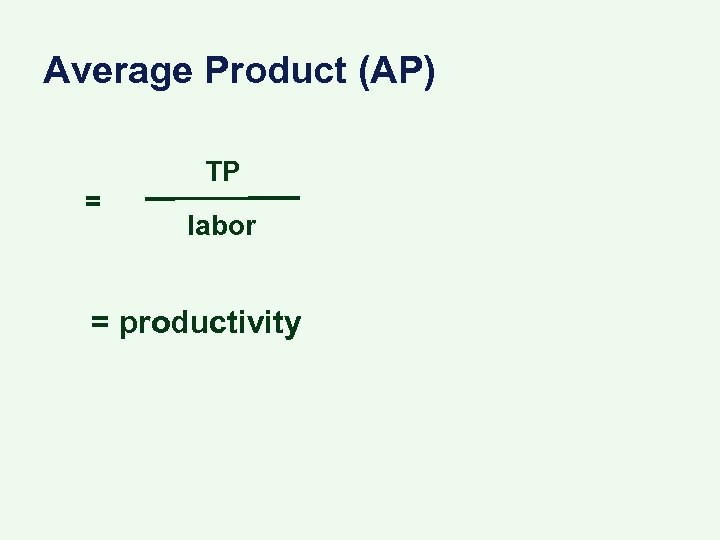Average Product (AP) = TP labor = productivity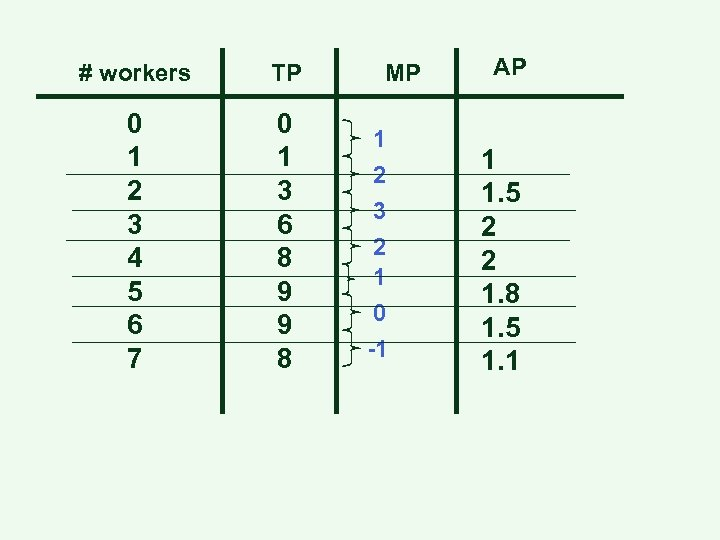# workers TP 0 1 2 3 4 5 6 7 0 1 3 6 8 9 9 8 MP 1 2 3 2 1 0 -1 AP 1 1. 5 2 2 1. 8 1. 5 1. 1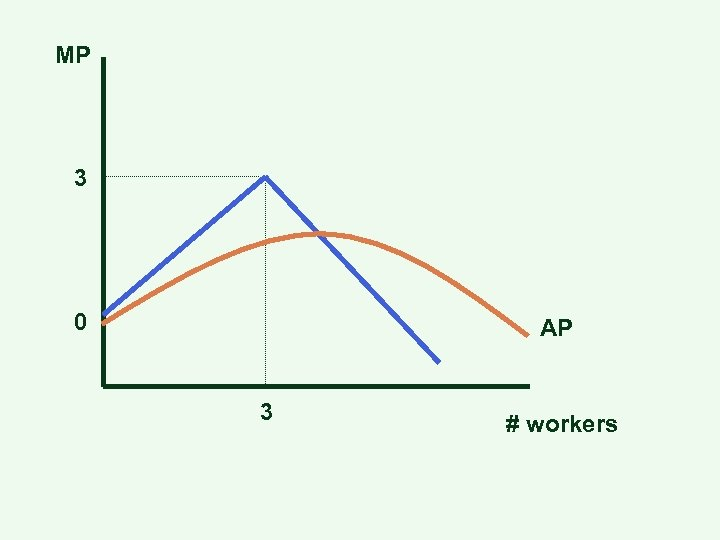MP 3 0 AP 3 # workers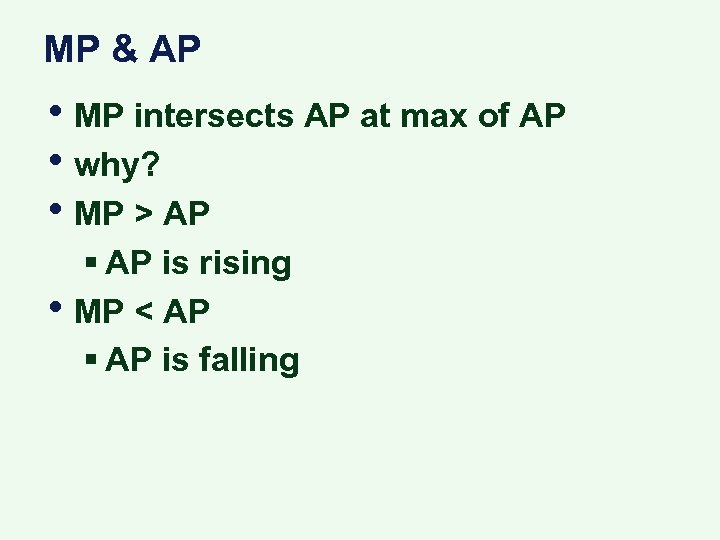MP & AP • MP intersects AP at max of AP • why? • MP > AP • § AP is rising MP < AP § AP is falling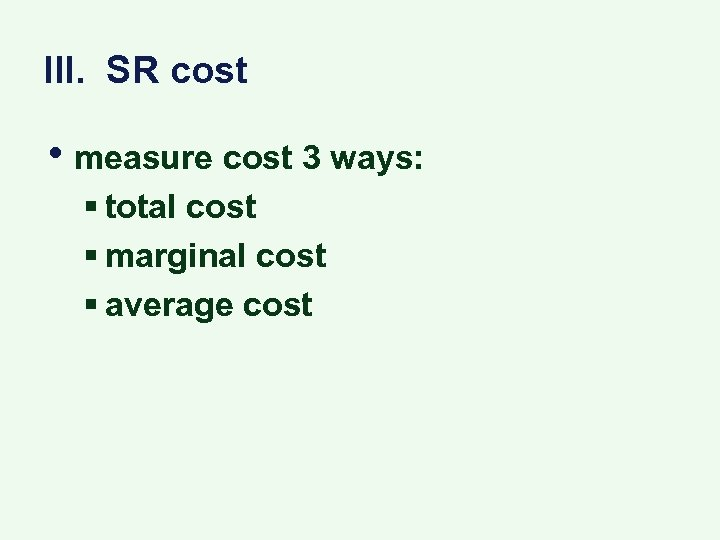III. SR cost • measure cost 3 ways: § total cost § marginal cost § average cost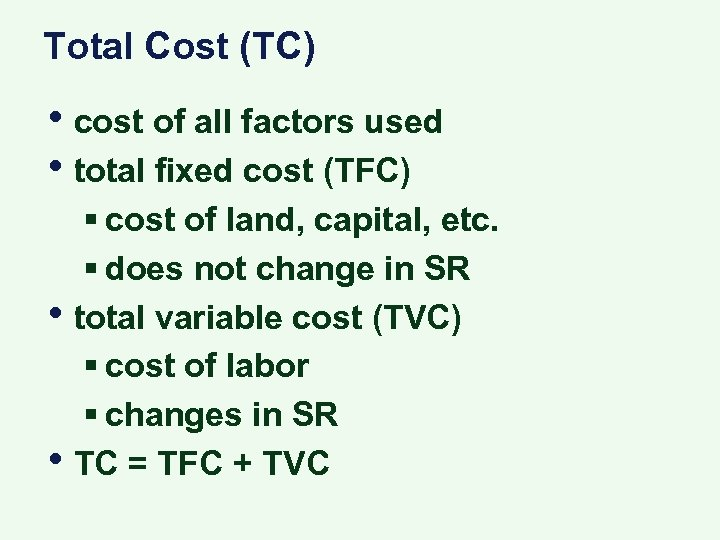Total Cost (TC) • cost of all factors used • total fixed cost (TFC) • • § cost of land, capital, etc. § does not change in SR total variable cost (TVC) § cost of labor § changes in SR TC = TFC + TVC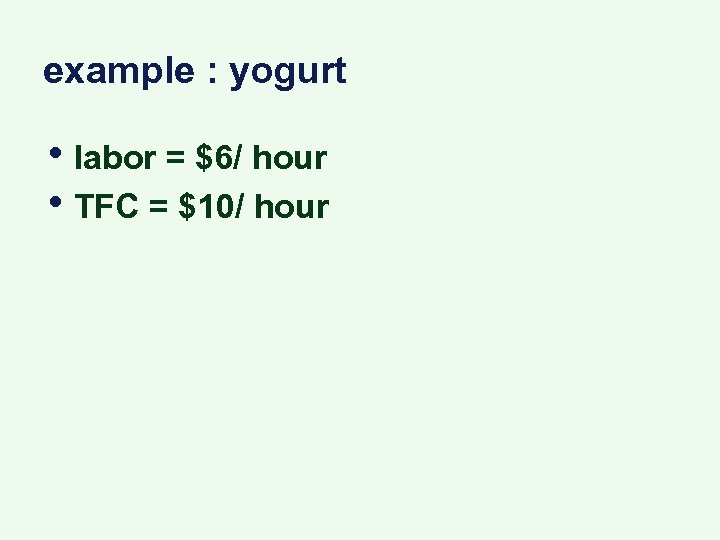example : yogurt • labor = \$6/ hour • TFC = \$10/ hour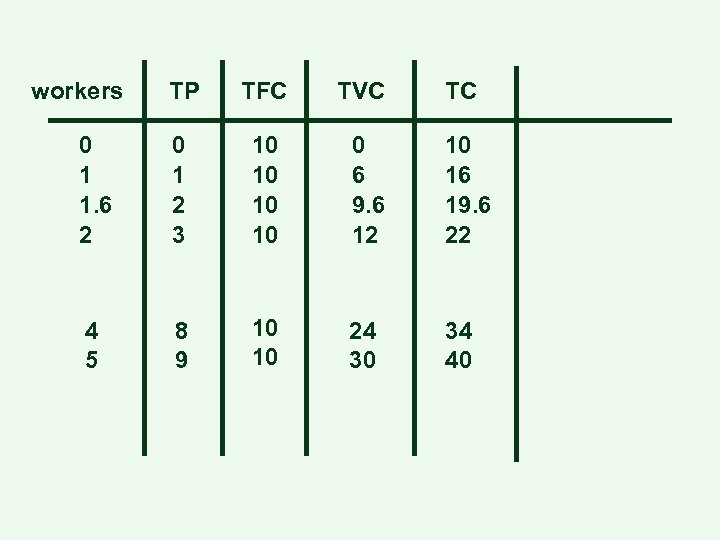workers TP TFC TVC TC 0 1 1. 6 2 0 1 2 3 10 10 0 6 9. 6 12 10 16 19. 6 22 4 5 8 9 10 10 24 30 34 40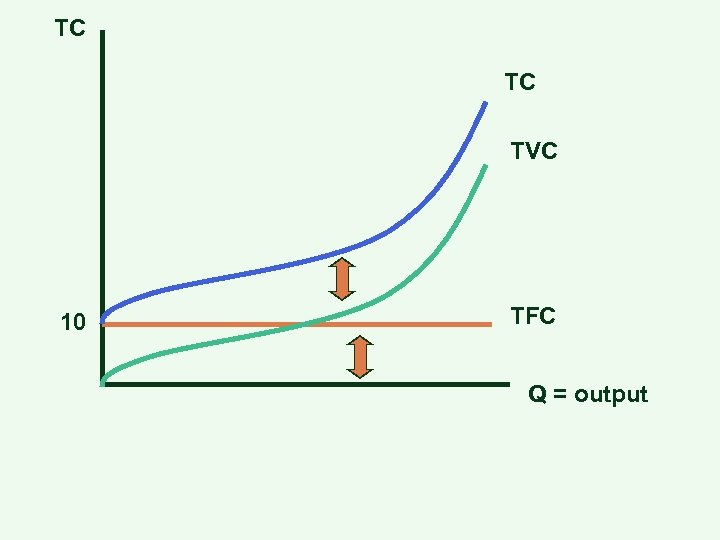TC TC TVC 10 TFC Q = output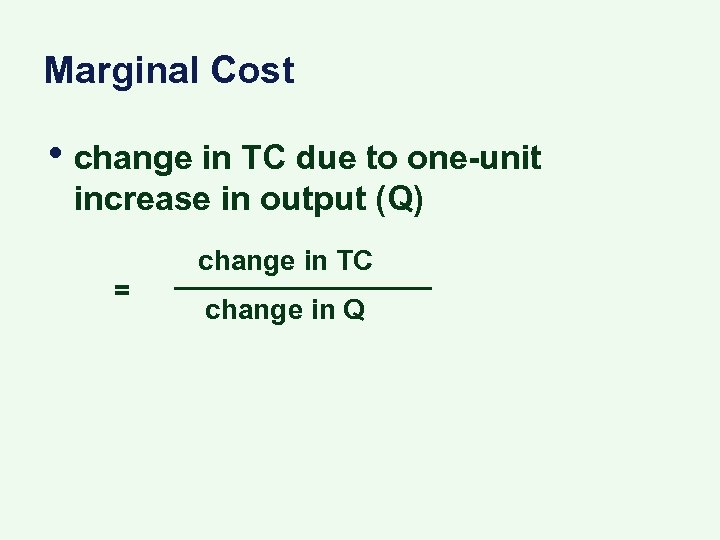Marginal Cost • change in TC due to one-unit increase in output (Q) = change in TC change in Q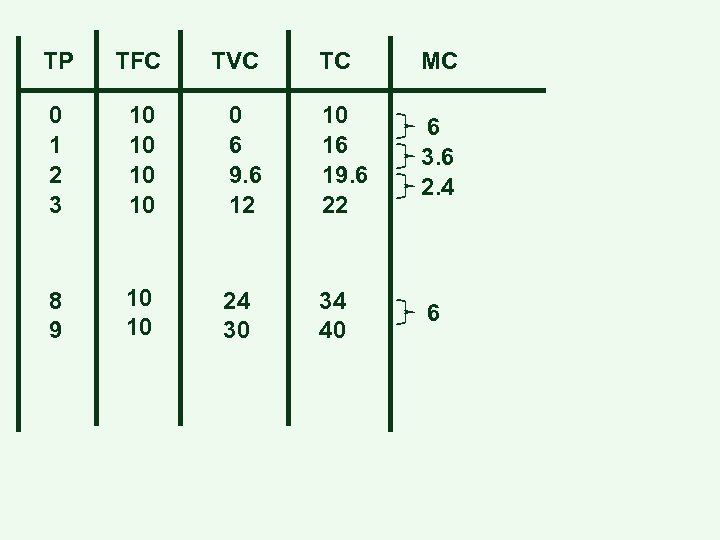TP TFC TVC TC MC 0 1 2 3 10 10 0 6 9. 6 12 10 16 19. 6 22 6 3. 6 2. 4 8 9 10 10 24 30 34 40 6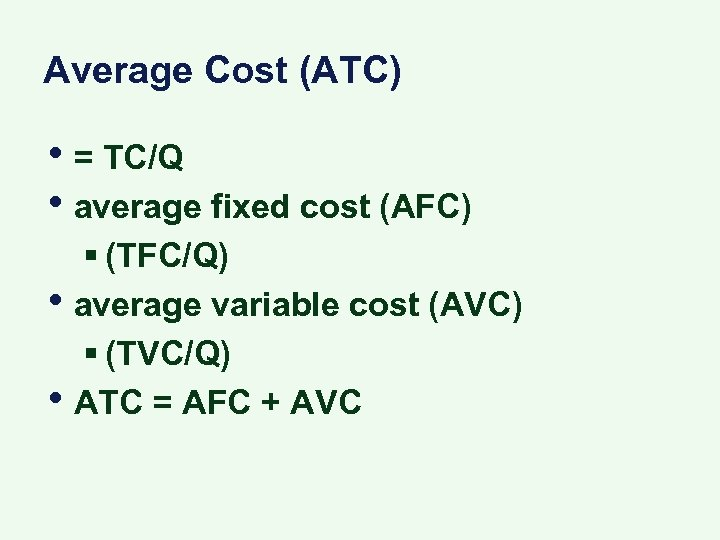Average Cost (ATC) • = TC/Q • average fixed cost (AFC) • • § (TFC/Q) average variable cost (AVC) § (TVC/Q) ATC = AFC + AVC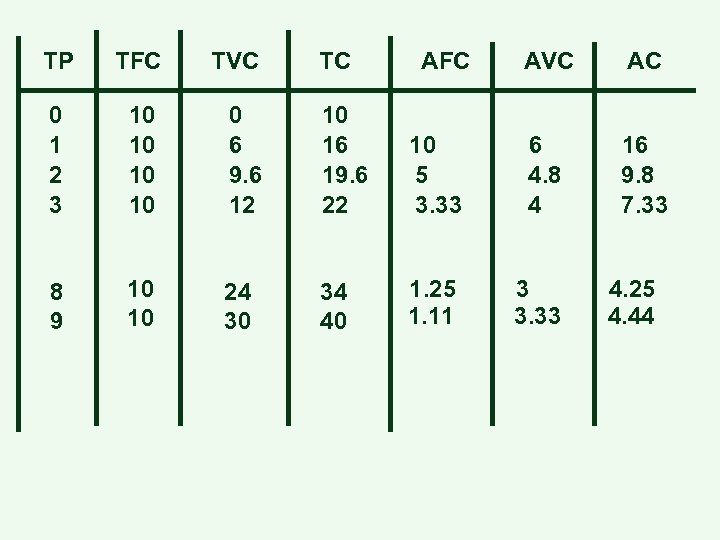TP TFC TVC TC 0 1 2 3 10 10 0 6 9. 6 12 10 16 19. 6 22 8 9 10 10 24 30 34 40 AFC AVC AC 10 5 3. 33 6 4. 8 4 16 9. 8 7. 33 1. 25 1. 11 3 3. 33 4. 25 4. 44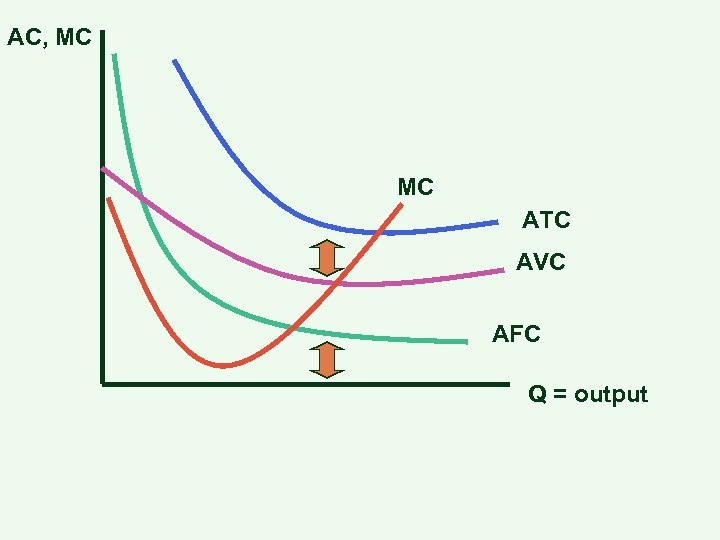AC, MC MC ATC AVC AFC Q = output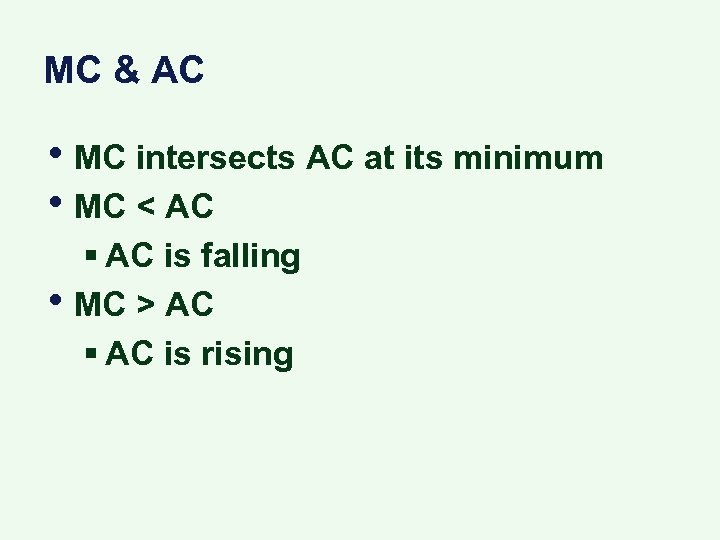MC & AC • MC intersects AC at its minimum • MC < AC • § AC is falling MC > AC § AC is rising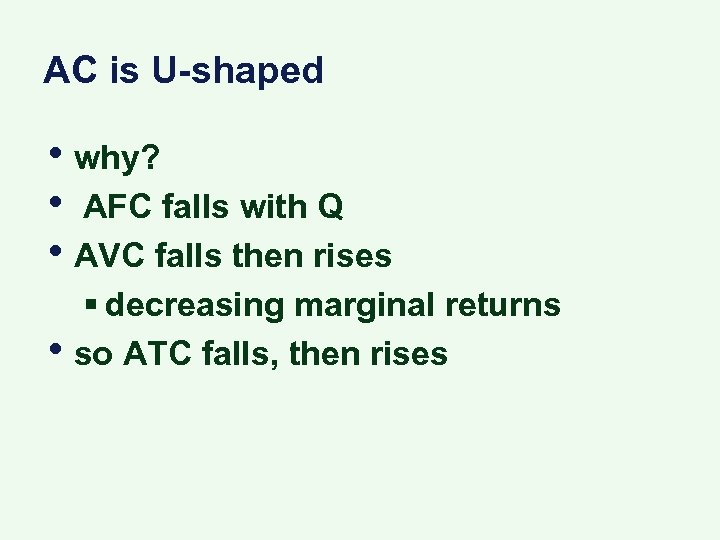AC is U-shaped • why? • AFC falls with Q • AVC falls then rises • § decreasing marginal returns so ATC falls, then rises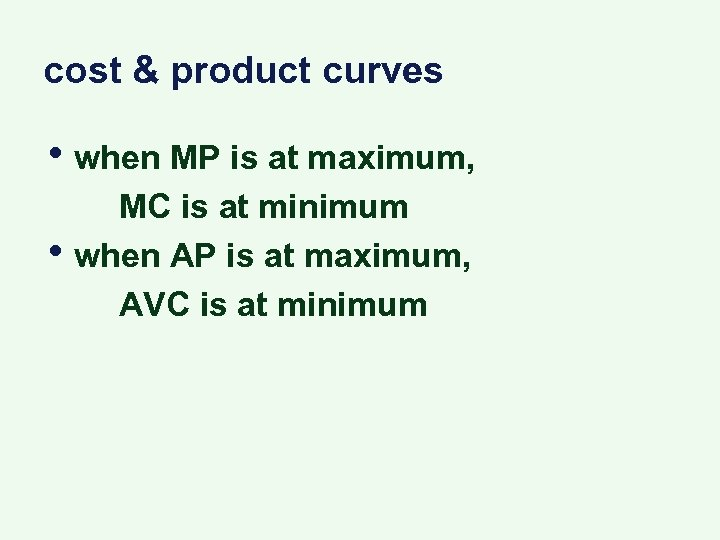cost & product curves • when MP is at maximum, • MC is at minimum when AP is at maximum, AVC is at minimum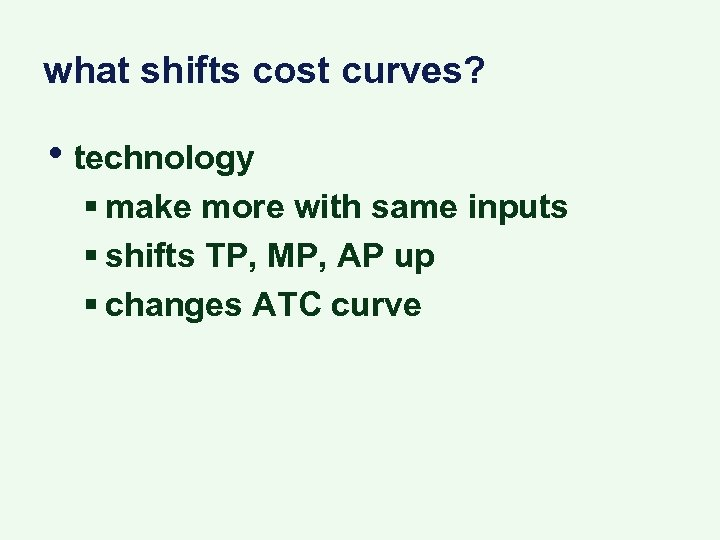what shifts cost curves? • technology § make more with same inputs § shifts TP, MP, AP up § changes ATC curve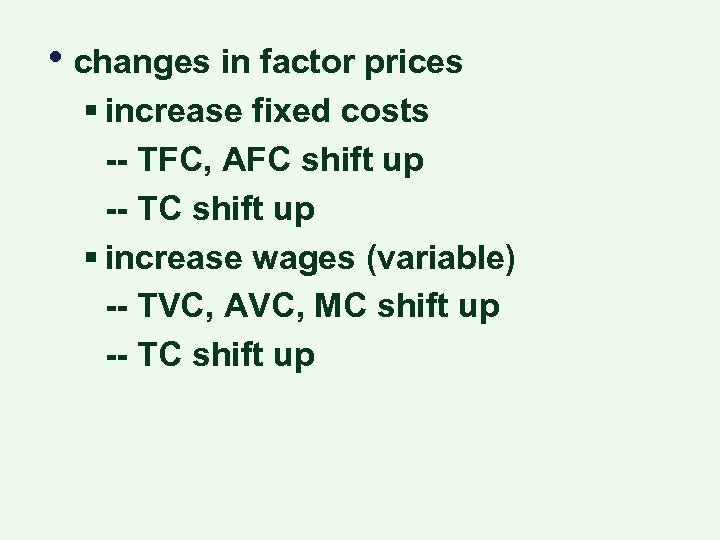• changes in factor prices § increase fixed costs -- TFC, AFC shift up -- TC shift up § increase wages (variable) -- TVC, AVC, MC shift up -- TC shift up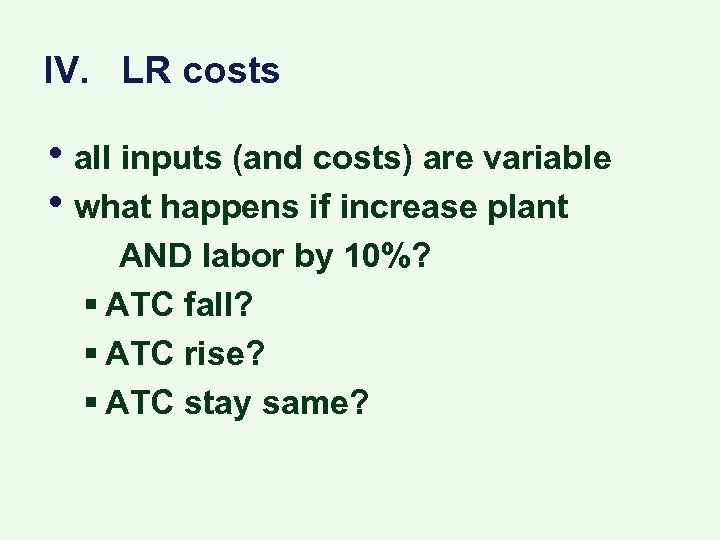IV. LR costs • all inputs (and costs) are variable • what happens if increase plant AND labor by 10%? § ATC fall? § ATC rise? § ATC stay same?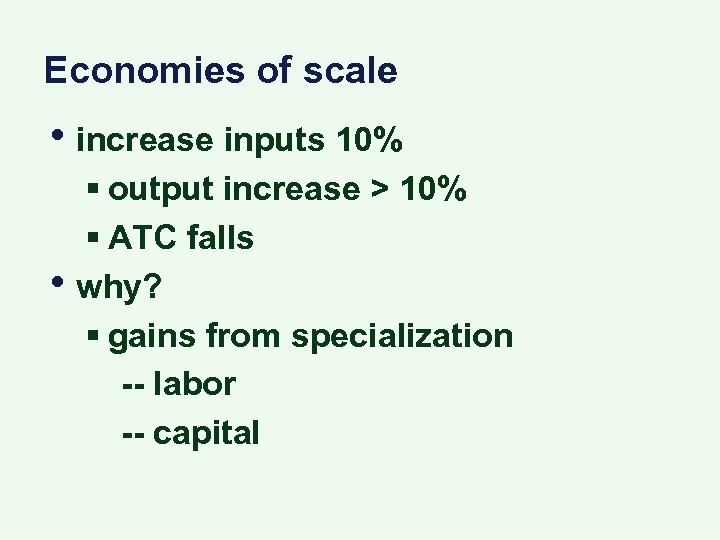Economies of scale • increase inputs 10% • § output increase > 10% § ATC falls why? § gains from specialization -- labor -- capital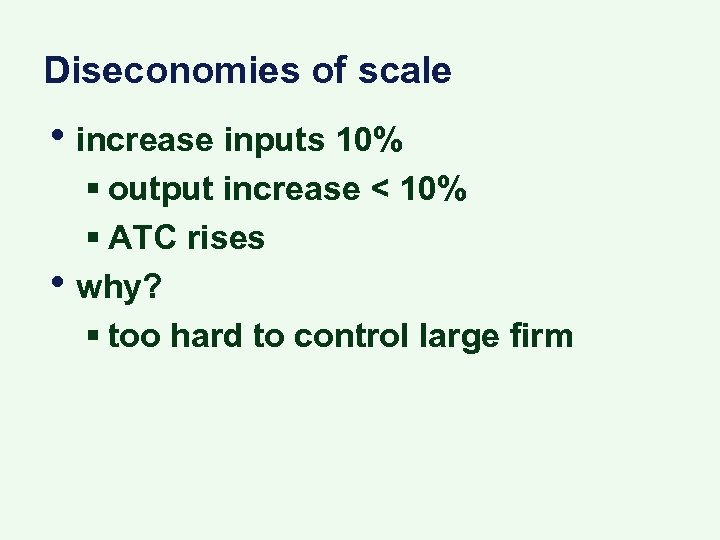Diseconomies of scale • increase inputs 10% • § output increase < 10% § ATC rises why? § too hard to control large firm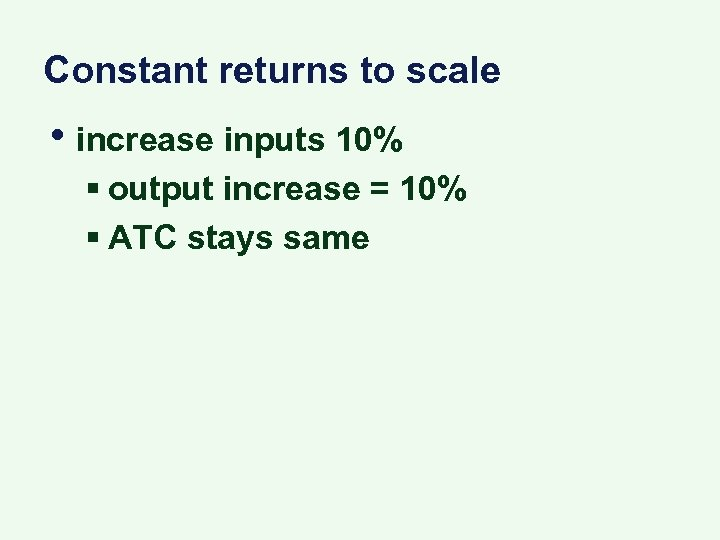Constant returns to scale • increase inputs 10% § output increase = 10% § ATC stays same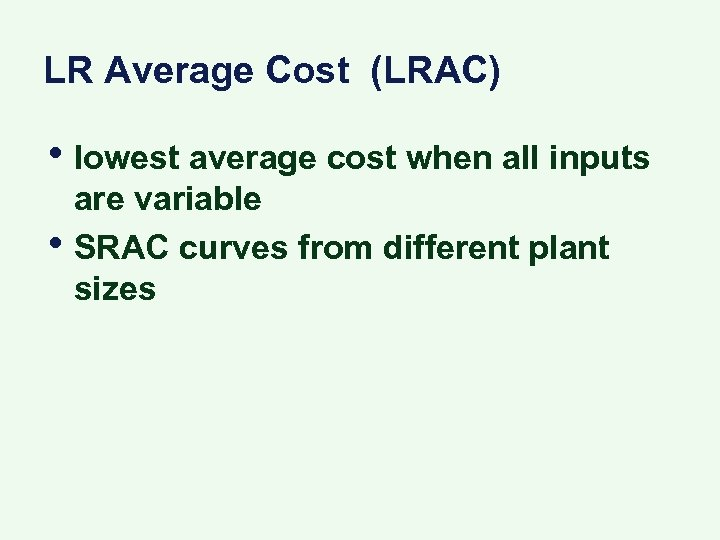LR Average Cost (LRAC) • lowest average cost when all inputs • are variable SRAC curves from different plant sizes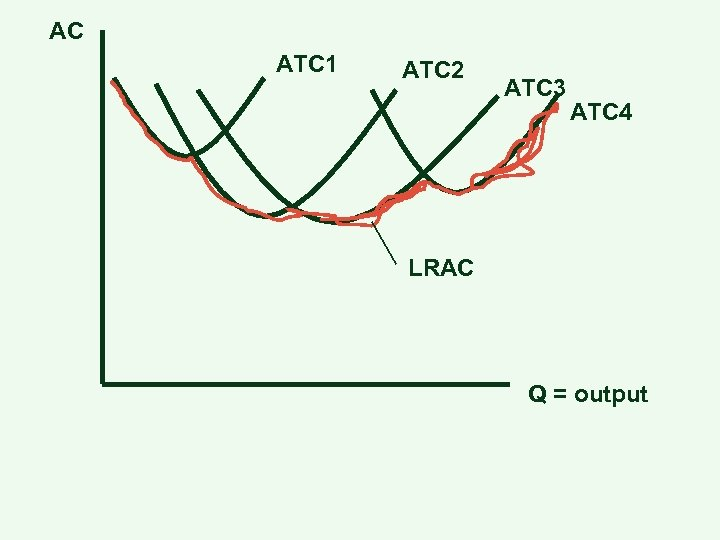AC ATC 1 ATC 2 ATC 3 ATC 4 LRAC Q = output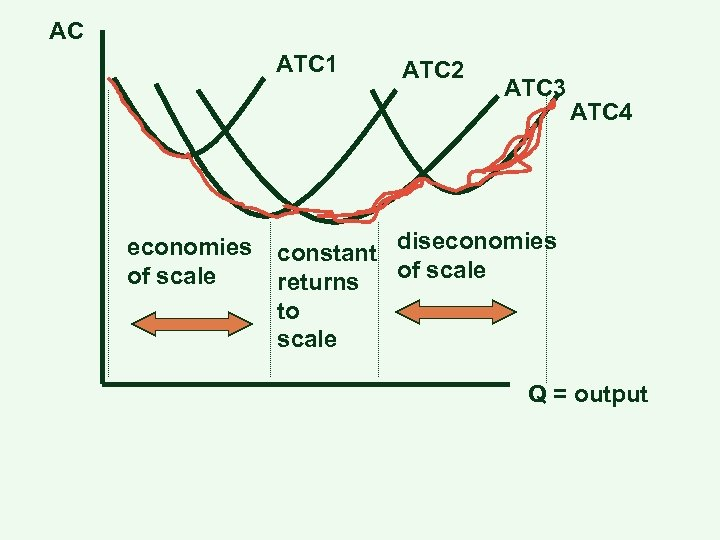AC ATC 1 economies of scale ATC 2 ATC 3 ATC 4 constant diseconomies of scale returns to scale Q = output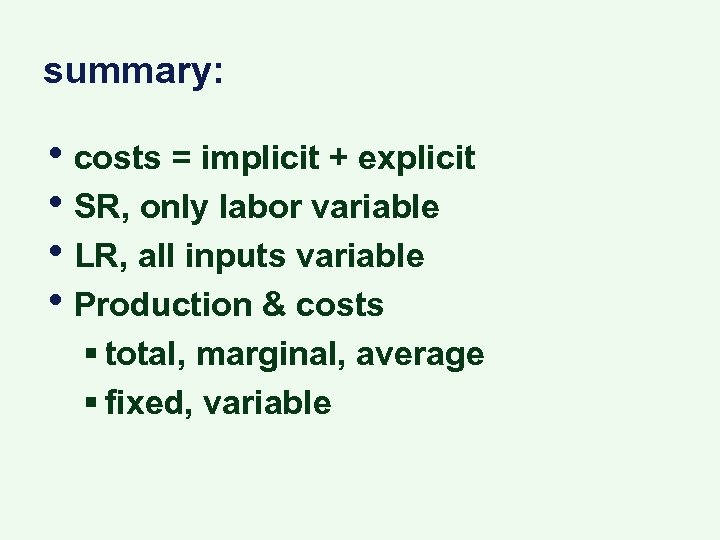summary: • costs = implicit + explicit • SR, only labor variable • LR, all inputs variable • Production & costs § total, marginal, average § fixed, variable